# Logistic Regression From Scratch With Python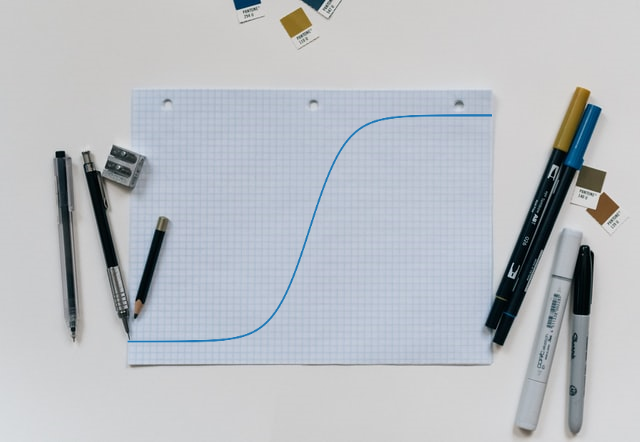This tutorial covers basic concepts of logistic regression. I will explain the process of creating a model right from hypothesis function to algorithm. We will also use plots for better visualization of inner workings of the model. At the end we will test our model for binary classification.

# Introduction

Unlike Linear Regression, Logistic Regression is used to solve classification problem like classifying email as spam or not spam. Don’t get confuse by the name ‘Regression’, Logistic Regression is a ‘Classification Algorithm’. If the value that we are trying to classify takes on only two values 0(negative/false) or 1 (positive/true) then we call it ‘Binary Classification’ and if there are more than two classes, then we call it ‘Multi Class Classification’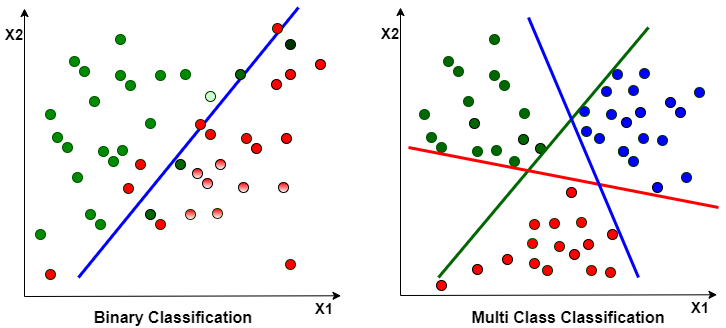We will create our own Logistic regression algorithm and build a classification model that estimates an applicant’s probability of admission based on Exam 1 and Exam 2 scores.

Logistic Regression is Supervised Learning Algorithm.

I am using same notation and example data used in Andrew Ng’s Machine Learning course

## Why Can’t We use Linear Regression For Classification

• We have already learned about Linear Regression, so you must be wondering why do we need Logistic Regression? Can’t we use Linear Regression to solve the classification problems?
• Remember that Linear Regression works well when there is linear relation between output and input values
• In classification our objective is to get discrete value like 0 or 1. But in case of Linear regression our objective is to get continuous value which can range outside 0 and 1. This is one of the problem, as we are trying to classify output as 0 or 1 but Linear Regression algorithm isn’t designed for predicting discrete values.
• So in order to get discrete output from Linear Regression we can use threshold value(0.5) to classify output. Classify anything above 0.5 as positive class(1) and anything below 0.5 as negative class(0).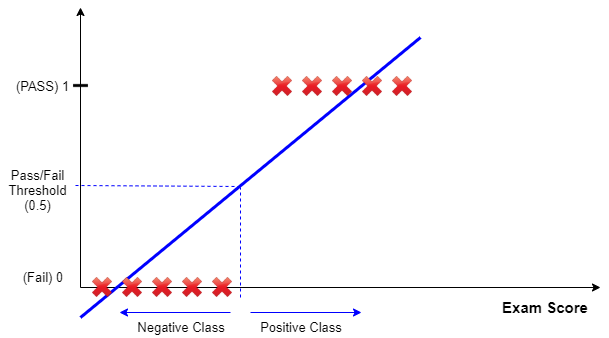• But this adjustment also do not work if there are outliers in dataset. Notice the slope of line in below figure and incorrect prediction.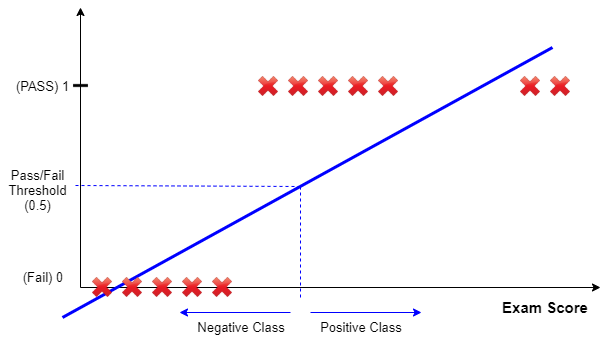• That’s why we can’t use Linear Regression to solve classification problem, and we need dedicated algorithm for it.

## Hypothesis Function

• Since our objective is to get discrete value(0 or 1) we will create a hypothesis function that will return values between 0 and 1
• Sigmoid function do exactly that, it maps the whole real number range between 0 and 1. It is also called as Logistic function.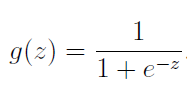• The term Sigmoid means ‘S-shaped’ and when plotted this function gives S-shaped curve. In below figure for given range of X values, Y values ranges from 0 to 1 only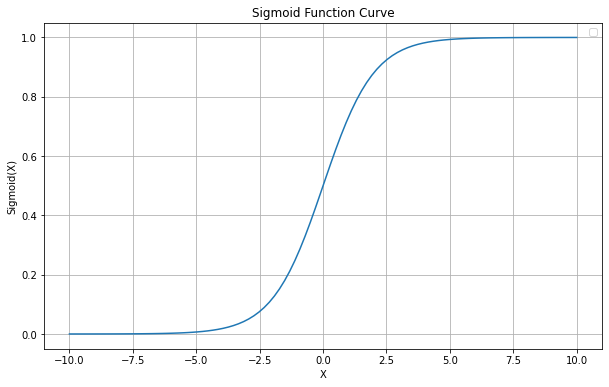• Remember hypothesis function for Linear Regression is
``````  h(x) = θ_0 + (θ_1*x_1)....(θ_n*x_n)
``````

We are going to use this function as input to our Sigmoid function to get the discrete values.

``````  z = θ_0 + (θ_1*x_1)....(θ_n*x_n)
``````
• Hypothesis function for Logistic Regression is
``````  h(x) = g(z) = g(θ_0 + (θ_1*x_1)....(θ_n*x_n))
``````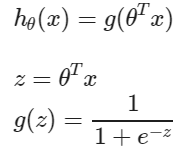• Basically we are using line function as input to sigmoid function in order to get discrete value from 0 to 1. The way our sigmoid function g(z) behaves is that, when its input is greater than or equal to zero, its output is greater than or equal to 0.5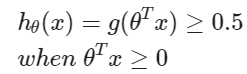• Since positive input results in positive class and negative input results in negative class, we can separate both the classes by setting the weighted sum of inputs to 0. i.e.

``````  z = θ_0 + (θ_1*x_1)....(θ_n*x_n) = 0
``````

## Decision Boundary

• Decision boundary separates the positive and negative class
• The decision boundary is the line that separates the area where y = 0 and where y = 1. It is created by our hypothesis function.
• As explained earlier decision boundary can be found by setting the weighted sum of inputs to 0
• Lets create a formula to find decision boundary for two feature(x and x1) dataset

``````  h(x) = θ_0 + (θ_1*x_1) + (θ_2*x_2) = 0
x_2 = -(θ_0 + (θ_1*x_1)) / θ_2
``````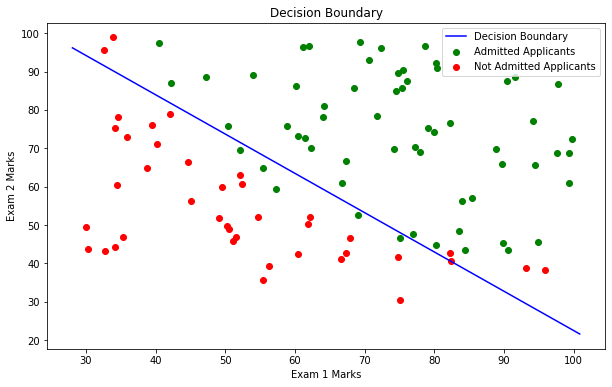Now we have our hypothesis function and decision boundary formula to classify the given data. Just like linear regression lets define a cost function to find the optimum values of theta parameters.

## Cost Function

It is obvious that to find the optimum values of theta parameters we have to try multiple values and then choose the best possible values based on how the predicted class match with given data. To do this we will create a cost function (J). Inner working of cost function is as below

• We will start with random values of θ_0 and θ_1
• We will execute the hypothesis function using theta values, to get the predicted values(0 or 1) for every training example
• Now we will compare our predicted discrete values with actual target values from training data.
• If our predicted value matches with actual value then our cost will be ‘0’ or else cost will be highly penalized.
• Logistic Regression cost function is as below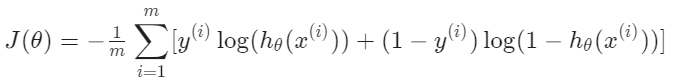• Vectorized implementation Of Logistic Regression cost function is as below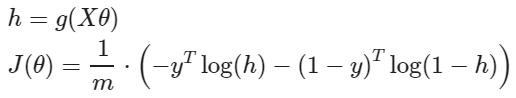• Just like linear regression logistic cost function is also ‘Convex function’
• So the optimum values of the theta are the one, for which we get minimum value of the cost.

Now we are going to use ‘Advanced Optimization Algorithm’ to find theta values.

## Advance Optimization Algorithm

• We are going to use fmin_tnc() function from ‘scipy’ library to find theta values
• This function will use cost function and gradient of the cost to find the optimum values of theta.
• Gradient of the cost is nothing but partial derivative of cost function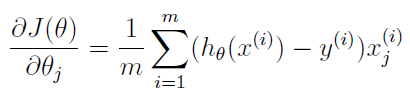• Note that while this gradient of cost looks identical to the linear regression gradient, the formula is actually different because linear and logistic regression have different definitions of h(x).

Enough of theory, now lets implement logistic regression algorithm using Python and create our classification model

# Python Code

Now we will implement the Logistic regression algorithm in Python and build a classification model that estimates an applicant’s probability of admission based on Exam 1 and Exam 2 scores.

## Notations used

• m = no of training examples (no of rows of feature matrix)
• n = no of features (no of columns of feature matrix)
• X’s = input variables / independent variables / features
• y’s = output variables / dependent variables / target/ labels

## Import Libraries

• pandas: Used for data manipulation and analysis
• numpy : Numpy is the core library for scientific computing in Python. It is used for working with arrays and matrices.
• matplotlib : It’s plotting library, and we are going to use it for data visualization
• scipy.optimize: Advance optimization library
``````import pandas as pd
import numpy as np
import matplotlib.pyplot as plt
import scipy.optimize as opt
``````

## Load The Data

• We are going to use ‘admission_basedon_exam_scores.csv’ CSV file
• File contains three columns Exam 1 marks, Exam 2 marks and Admission status
``````df = pd.read_csv('https://raw.githubusercontent.com/satishgunjal/datasets/master/admission_basedon_exam_scores.csv')
m, n = df.shape
print('Number of training examples m = ', m)
print('Number of features n = ', n - 1) # Not counting the 'Label: Admission status'
df.sample(5) # Show random 5 training examples
``````
``````Number of training examples m =  100
Number of features n =  2
``````
Exam 1 marks Exam 2 marks Admission status
38 74.789253 41.573415 0
45 62.222676 52.060992 0
19 76.978784 47.575964 1
3 60.182599 86.308552 1
66 40.457551 97.535185 1

## Data Understanding

• There are total 100 training examples (m= 100 or 100 no of rows)
• There are two features Exam 1 marks and Exam 2 marks
• Label column contains application status. Where ‘1’ means admitted and ‘0’ means not admitted
• Total no of features (n) = 2 (Later we will add column of ones(x_0) to make it 3)

## Data Visualization

To plot the data of admitted and not admitted applicants, we need to first create separate data frame for each class(admitted/not-admitted)

``````df_admitted = df[df['Admission status'] == 1]
print('Dimension of df_admitted= ', df_admitted.shape)
df_admitted.sample(5)
``````
``````Dimension of df_admitted=  (60, 3)
``````
Exam 1 marks Exam 2 marks Admission status
81 94.834507 45.694307 1
46 77.193035 70.458200 1
88 78.635424 96.647427 1
6 61.106665 96.511426 1
4 79.032736 75.344376 1
``````df_notadmitted = df[df['Admission status'] == 0]
print('Dimension of df_notadmitted= ', df_notadmitted.shape)
df_notadmitted.sample(5)
``````
``````Dimension of df_notadmitted=  (40, 3)
``````
Exam 1 marks Exam 2 marks Admission status
22 50.534788 48.855812 0
65 66.560894 41.092098 0
63 30.058822 49.592974 0
43 82.368754 40.618255 0
39 34.183640 75.237720 0

Now lets plot the scatter plot for admitted and not admitted students

``````plt.figure(figsize = (10,6))
plt.scatter(df_admitted['Exam 1 marks'], df_admitted['Exam 2 marks'], color='green', label='Admitted Applicants')
plt.scatter(df_notadmitted['Exam 1 marks'], df_notadmitted['Exam 2 marks'], color='red', label='Not Admitted Applicants')
plt.xlabel('Exam 1 Marks')
plt.ylabel('Exam 2 Marks')
plt.legend()
plt.title('Admitted Vs Not Admitted Applicants')
``````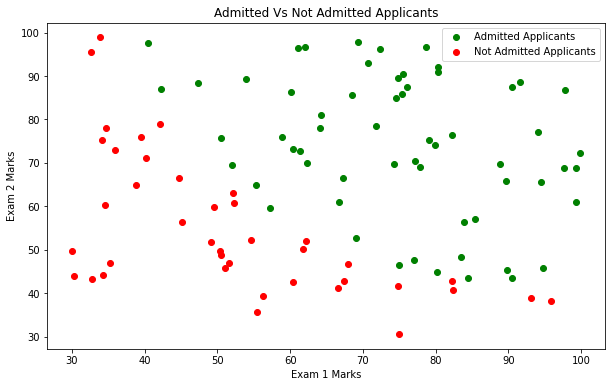## Compute Cost Using Cost Function

### Create Feature Matrix X and Label Vector y

If you want to know the reason behind adding column of ones to feature matrix, please refer ‘Vector Representation Of Hypothesis Function’ in Univariate Linear Regression

``````# Get feature columns from dataframe
X = df.iloc[:, 0:2]
#Add column of ones (intercept term)
X = np.hstack((np.ones((m,1)),X))
# Now X is numpy array of 2 dimension
print("Dimension of feature matric X = ", X.shape, '\n')

y = df.iloc[:, -1]
# First 5 records training examples with labels
for i in range(5):
print('x =', X[i, ], ', y =', y[i])
``````
``````Dimension of feature matric X =  (100, 3)

x = [ 1.         34.62365962 78.02469282] , y = 0
x = [ 1.         30.28671077 43.89499752] , y = 0
x = [ 1.         35.84740877 72.90219803] , y = 0
x = [ 1.         60.18259939 86.3085521 ] , y = 1
x = [ 1.         79.03273605 75.34437644] , y = 1
``````

Lets also initialize the theta values with 0

``````theta = np.zeros(n)
theta
``````
``````array([0., 0., 0.])
``````

### Create Sigmoid Function

``````def sigmoid(z):
"""
To convert continuous value into a range of 0 to 1

I/P
----------
z : Continuous value

O/P
-------
Value in range between 0 to 1.
"""
g = 1 / (1 + np.exp(-z))
return g
``````

### Create Cost And Gradient Function

We are using vector implementation of cost and gradient function formula’s for better performance

``````def cost_function(theta, X, y):
"""
Compute cost for logistic regression.

I/P
----------
X : 2D array where each row represent the training example and each column represent the feature ndarray. Dimension(m x n)
m= number of training examples
n= number of features (including X_0 column of ones)
y : 1D array of labels/target value for each traing example. dimension(1 x m)

theta : 1D array of fitting parameters or weights. Dimension (1 x n)

O/P
-------
J : The cost of using theta as the parameter for linear regression to fit the data points in X and y.
"""
m, n = X.shape
x_dot_theta = X.dot(theta)

J = 1.0 / m * (-y.T.dot(np.log(sigmoid(x_dot_theta))) - (1 - y).T.dot(np.log(1 - sigmoid(x_dot_theta))))

return J
``````
``````def gradient(theta, X, y):
"""
Compute gradient for logistic regression.

I/P
----------
X : 2D array where each row represent the training example and each column represent the feature ndarray. Dimension(m x n)
m= number of training examples
n= number of features (including X_0 column of ones)
y : 1D array of labels/target value for each traing example. dimension(1 x m)

theta : 1D array of fitting parameters or weights. Dimension (1 x n)

O/P
-------
grad: (numpy array)The gradient of the cost with respect to the parameters theta
"""
m, n = X.shape
x_dot_theta = X.dot(theta)

grad = 1.0 / m * (sigmoid(x_dot_theta) - y).T.dot(X)

return grad
``````

Testing the cost_function() using initial values

``````cost = cost_function(theta, X, y)
print ('Cost at initial theta (zeros):', cost)

grad = gradient(theta, X, y)
print ('Gradient at initial theta (zeros):', grad)
``````
``````Cost at initial theta (zeros): 0.6931471805599453
Gradient at initial theta (zeros): [ -0.1        -12.00921659 -11.26284221]
``````

## Using Advance Optimization Technique To Find Theta Values

• Remember in Univariate Linear Regression tutorial we have created a gradient descent function to find the theta values.
• But here we are going use fmin_tnc function from the scipy library
• This process is same as using ‘fit’ method from sklearn library. Because here we are trying to optimize our cost function in order to find the best possible parameter(theta) values
• fmin_tnc function takes four arguments:
• func: Cost function to minimize
• fprime: Gradient for the function defined by ‘func’
• x0: initial values for the parameters(theta) that we want to find
• args: feature and label values
``````theta, nfeval, rc = opt.fmin_tnc(func=cost_function, fprime= gradient, x0=theta, args=(X, y))

cost = cost_function(theta, X, y)
print ('Cost at theta found by fminunc:', cost)
print ('theta:', theta)
``````
``````Cost at theta found by fminunc: 0.20349770158947436
theta: [-25.16131865   0.20623159   0.20147149]
``````

## Visualization

Let’s plot decision boundary to cross-check the accuracy of our model

``````# Lets calculate the X and Y values using Decision Boundary formula

# For ploting a line we just need 2 points. Here I am taking 'min' and 'max' value as my two X points
x_values = [min(X[:, 1]), np.max(X[:, 2])]
y_values = - (theta + np.dot(theta, x_values)) / theta

plt.figure(figsize = (10,6))
plt.scatter(df_admitted['Exam 1 marks'], df_admitted['Exam 2 marks'], color='green', label='Admitted Applicants')
plt.scatter(df_notadmitted['Exam 1 marks'], df_notadmitted['Exam 2 marks'], color='red', label='Not Admitted Applicants')
plt.xlabel('Exam 1 Marks')
plt.ylabel('Exam 2 Marks')

plt.plot(x_values, y_values, color='blue', label='Decision Boundary')
plt.legend()
plt.title('Decision Boundary')
``````## Model Testing

• Question: Predict an admission probability for applicant with scores 45 in Exam 1 and 85 in Exam 2
• We can use our hypothesis function for prediction h(x) = g(z) = g(Xθ)
``````input_data = np.array([1, 45, 85]) # Note the intercept term '1' in array
prob = sigmoid(np.dot(input_data, theta))
print ('Admission probability for applicant with scores 45 in Exam 1 and 85 in Exam 2 is =', prob)
``````
``````Admission probability for applicant with scores 45 in Exam 1 and 85 in Exam 2 is = 0.7762906229081791
``````

Let’s create a function for prediction on our logistic model. Instead of predicting the probability between 0 and 1, this function will use threshold value of 0.5 to predict the discrete value. 1 when probability >= 0.5 else 0

``````def predict(theta, X):
"""
Predict the class between 0 and 1 using learned logistic regression parameters theta.
Using threshold value 0.5 to convert probability value to class value

I/P
----------
X : 2D array where each row represent the training example and each column represent the feature ndarray. Dimension(m x n)
m= number of training examples
n= number of features (including X_0 column of ones)

theta : 1D array of fitting parameters or weights. Dimension (1 x n)

O/P
-------
Class type based on threshold
"""
p = sigmoid(X.dot(theta)) >= 0.5
return p.astype(int)
``````

### Accuracy Of Model

``````predictedValue = pd.DataFrame(predict(theta, X), columns=['Predicted Admission status']) # Create new dataframe of column'Predicted Price'
actualAdmissionStatus = pd.DataFrame(y, columns=['Admission status'])
df_actual_vs_predicted = pd.concat([actualAdmissionStatus,predictedValue],axis =1)
df_actual_vs_predicted.T
``````
0 1 2 3 4 5 6 7 8 9 10 11 12 13 14 15 16 17 18 19 20 21 22 23 24 25 26 27 28 29 30 31 32 33 34 35 36 37 38 39 ... 60 61 62 63 64 65 66 67 68 69 70 71 72 73 74 75 76 77 78 79 80 81 82 83 84 85 86 87 88 89 90 91 92 93 94 95 96 97 98 99
Admission status 0 0 0 1 1 0 1 1 1 1 0 0 1 1 0 1 1 0 1 1 0 1 0 0 1 1 1 0 0 0 1 1 0 1 0 0 0 1 0 0 ... 1 0 0 0 0 0 1 0 1 1 0 1 1 1 1 1 1 1 0 0 1 1 1 1 1 1 0 1 1 0 1 1 0 1 1 1 1 1 1 1
Predicted Admission status 0 0 0 1 1 0 1 0 1 1 1 0 1 1 0 1 0 0 1 1 0 1 0 0 1 1 1 1 0 0 1 1 0 0 0 0 1 1 0 0 ... 1 0 0 0 0 0 1 0 1 1 0 1 1 1 1 1 1 1 0 1 1 1 1 0 1 1 0 1 1 0 1 1 0 1 1 1 1 1 0 1

2 rows × 100 columns

``````p = predict(theta, X)
print ('Accuracy:', np.mean(p == y) * 100 )
``````
``````Accuracy: 89.0
``````

## Conclusion

This conludes our logistic regression. We have covered hypothesis function, cost function and cost function optimization using advance optimization technique. We have also tested our model for binary classification using exam test data. I will also create one more study using Sklearn logistic regression model.

## Learning Path for DP-900 Microsoft Azure Data Fundamentals Certification

8 minute read

Learning path to gain necessary skills and to clear the Azure Data Fundamentals Certification. This certification is intended for candidates beginning to wor...

## Learning Path for AI-900 Microsoft Azure AI Fundamentals Certification

7 minute read

Learning path to gain necessary skills and to clear the Azure AI Fundamentals Certification. This certification is intended for candidates with both technica...

## ANN Model to Classify Images

12 minute read

In this guide we are going to create and train the neural network model to classify the clothing images. We will use TensorFlow deep learning framework along...

## Introduction to NLP

8 minute read

In short NLP is an AI technique used to do text analysis. Whenever we have lots of text data to analyze we can use NLP. Apart from text analysis, NLP also us...

## K Fold Cross Validation

14 minute read

There are multiple ways to split the data for model training and testing, in this article we are going to cover K Fold and Stratified K Fold cross validation...

## K-Means Clustering

13 minute read

K-Means clustering is most commonly used unsupervised learning algorithm to find groups in unlabeled data. Here K represents the number of groups or clusters...

## Time Series Analysis and Forecasting

10 minute read

Any data recorded with some fixed interval of time is called as time series data. This fixed interval can be hourly, daily, monthly or yearly. Objective of t...

## Support Vector Machines

9 minute read

Support vector machines is one of the most powerful ‘Black Box’ machine learning algorithm. It belongs to the family of supervised learning algorithm. Used t...

## Random Forest

12 minute read

Random forest is supervised learning algorithm and can be used to solve classification and regression problems. Unlike decision tree random forest fits multi...

## Decision Tree

13 minute read

Decision tree explained using classification and regression example. The objective of decision tree is to split the data in such a way that at the end we hav...

## Agile Scrum Framework

7 minute read

This tutorial covers basic Agile principles and use of Scrum framework in software development projects.

## Underfitting & Overfitting

2 minute read

Main objective of any machine learning model is to generalize the learning based on training data, so that it will be able to do predictions accurately on un...

## Multiclass Logistic Regression Using Sklearn

6 minute read

In this study we are going to use the Linear Model from Sklearn library to perform Multi class Logistic Regression. We are going to use handwritten digit’s d...

## Binary Logistic Regression Using Sklearn

6 minute read

In this tutorial we are going to use the Logistic Model from Sklearn library. We are also going to use the same test data used in Logistic Regression From Sc...

## Logistic Regression From Scratch With Python

13 minute read

This tutorial covers basic concepts of logistic regression. I will explain the process of creating a model right from hypothesis function to algorithm. We wi...

## Train Test Split

3 minute read

In this tutorial we are going to study about train, test data split. We will use sklearn library to do the data split.

## One Hot Encoding

11 minute read

In this tutorial we are going to study about One Hot Encoding. We will also use pandas and sklearn libraries to convert categorical data into numeric data.

## Multivariate Linear Regression Using Scikit Learn

8 minute read

In this tutorial we are going to use the Linear Models from Sklearn library. Scikit-learn is one of the most popular open source machine learning library for...

## Univariate Linear Regression Using Scikit Learn

7 minute read

In this tutorial we are going to use the Linear Models from Sklearn library. Scikit-learn is one of the most popular open source machine learning library for...

## Multivariate Linear Regression From Scratch With Python

10 minute read

In this tutorial we are going to cover linear regression with multiple input variables. We are going to use same model that we have created in Univariate Lin...

## Univariate Linear Regression From Scratch With Python

13 minute read

This tutorial covers basic concepts of linear regression. I will explain the process of creating a model right from hypothesis function to gradient descent a...

## Machine Learning Introduction And Learning Plan

4 minute read

In this tutorial we will see the brief introduction of Machine Learning and preferred learning plan for beginners

Back to top ↑